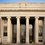[Challenge] Logarithmic Limit Barrage

Compute $\lim\limits_{n\to\infty}\left(\sqrt[n]{\log\left|1+\left(\dfrac{1}{n\cdot\log\left(n\right)}\right)^k\right|}\right).$

Source: A friend of mineNote by Ahaan Rungta
6 years, 4 months ago

This discussion board is a place to discuss our Daily Challenges and the math and science related to those challenges. Explanations are more than just a solution — they should explain the steps and thinking strategies that you used to obtain the solution. Comments should further the discussion of math and science.

When posting on Brilliant:

• Use the emojis to react to an explanation, whether you're congratulating a job well done , or just really confused .
• Ask specific questions about the challenge or the steps in somebody's explanation. Well-posed questions can add a lot to the discussion, but posting "I don't understand!" doesn't help anyone.
• Try to contribute something new to the discussion, whether it is an extension, generalization or other idea related to the challenge.
• Stay on topic — we're all here to learn more about math and science, not to hear about your favorite get-rich-quick scheme or current world events.

MarkdownAppears as
*italics* or _italics_ italics
**bold** or __bold__ bold

- bulleted
- list

• bulleted
• list

1. numbered
2. list

1. numbered
2. list
Note: you must add a full line of space before and after lists for them to show up correctly
paragraph 1

paragraph 2

paragraph 1

paragraph 2

> This is a quote
This is a quote
# I indented these lines
# 4 spaces, and now they show
# up as a code block.

print "hello world"
# I indented these lines
# 4 spaces, and now they show
# up as a code block.

print "hello world"
MathAppears as
Remember to wrap math in $$...$$ or $...$ to ensure proper formatting.
2 \times 3 $2 \times 3$
2^{34} $2^{34}$
a_{i-1} $a_{i-1}$
\frac{2}{3} $\frac{2}{3}$
\sqrt{2} $\sqrt{2}$
\sum_{i=1}^3 $\sum_{i=1}^3$
\sin \theta $\sin \theta$
\boxed{123} $\boxed{123}$

Sort by:

This actually turned out easier than I thought, thanks to Art of Problem Solving user aziiri. This was his solution: $\forall\ x\geq 0\ :\ x- \frac{x^2}{2}\leq \ln(1+x)\leq x.$ Apply to get that the limit equals $\boxed {1}$ for any real number $k$.

- 6 years, 4 months ago

Yep, that's me. Note that even if we replace $k$ with $-n$ we still get $1$, because the n-th degree root tends towards one a lot faster that the logarithm and the identity function.

- 6 years, 4 months ago

Also you can take ln(nat log) and then evaluate it, it would come ln(L)=0 where 'L' is the limit.

- 6 years, 4 months ago

what is nat in ln(nat log)?

- 6 years, 4 months ago

'nat' is natural log(base e), Budha.

- 6 years, 4 months ago

Can u provide this "Art of Problem Solving" for me ?! is it a book or what ?!

- 6 years, 4 months ago

It is a website: www.artofproblemsolving.com.

- 6 years, 4 months ago

You made my day , Thx !

- 6 years, 4 months ago

Can you tell me the ans Ahaan?

- 6 years, 4 months ago

Numerically, I have found the answer to be $1$ for all values of $k$.

- 6 years, 4 months ago

Yeah, me too.

- 6 years, 4 months ago

it is 1 , m sure :)

- 6 years, 4 months ago

1

- 6 years, 4 months ago

I've never been an expert on limits but i think its 0... if n is realy large, 1/(nlogn) will be 0 pretty much, regardless of the k... then it becomes the nth root of the square root of log(1) which is the nth root of 0 which is 0.. sure theres a way better way of doing this lol

- 6 years, 4 months ago

0

- 6 years, 4 months ago

Its 0

- 6 years, 4 months ago

$e^{\frac{-1}{2}}$

- 6 years, 4 months ago

sin33 in surd form

- 6 years, 4 months ago